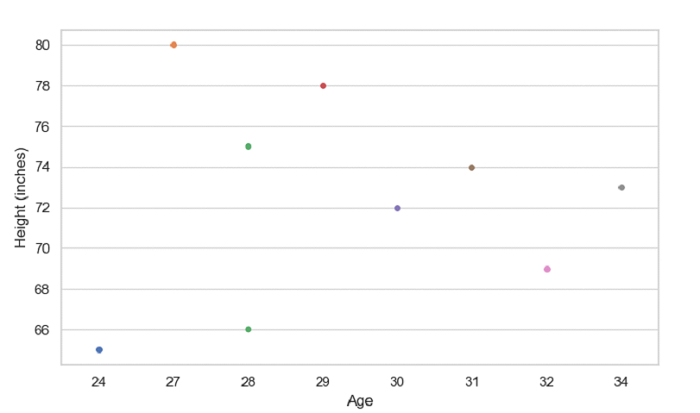# Create a Swarm Plot with SeaBorn – Python Pandas

Swarm Plot in Seaborn is used to draw a categorical scatterplot with non-overlapping points. The seaborn.swarmplot() is used for this.

Let’s say the following is our dataset in the form of a CSV file − Cricketers.csv

At first, import the required 3 libraries −

import seaborn as sb
import pandas as pd
import matplotlib.pyplot as plt

Load data from a CSV file into a Pandas DataFrame −

dataFrame = pd.read_csv("C:\Users\amit_\Desktop\Cricketers.csv")


Plotting swarm plot with Age and Height (inches) −

sb.swarmplot(x = dataFrame["Age"], y = dataFrame["Height"], data=dataFrame)

## Example

Following is the code −

import seaborn as sb
import pandas as pd
import matplotlib.pyplot as plt

# Load data from a CSV file into a Pandas DataFrame:

sb.set_theme(style="whitegrid")

# plotting swarm plot with Age and Height (inches)
sb.swarmplot(x = dataFrame["Age"], y = dataFrame["Height"], data=dataFrame)

# set label
plt.ylabel("Height (inches)")

# display
plt.show()

## Output

This will produce the following output −